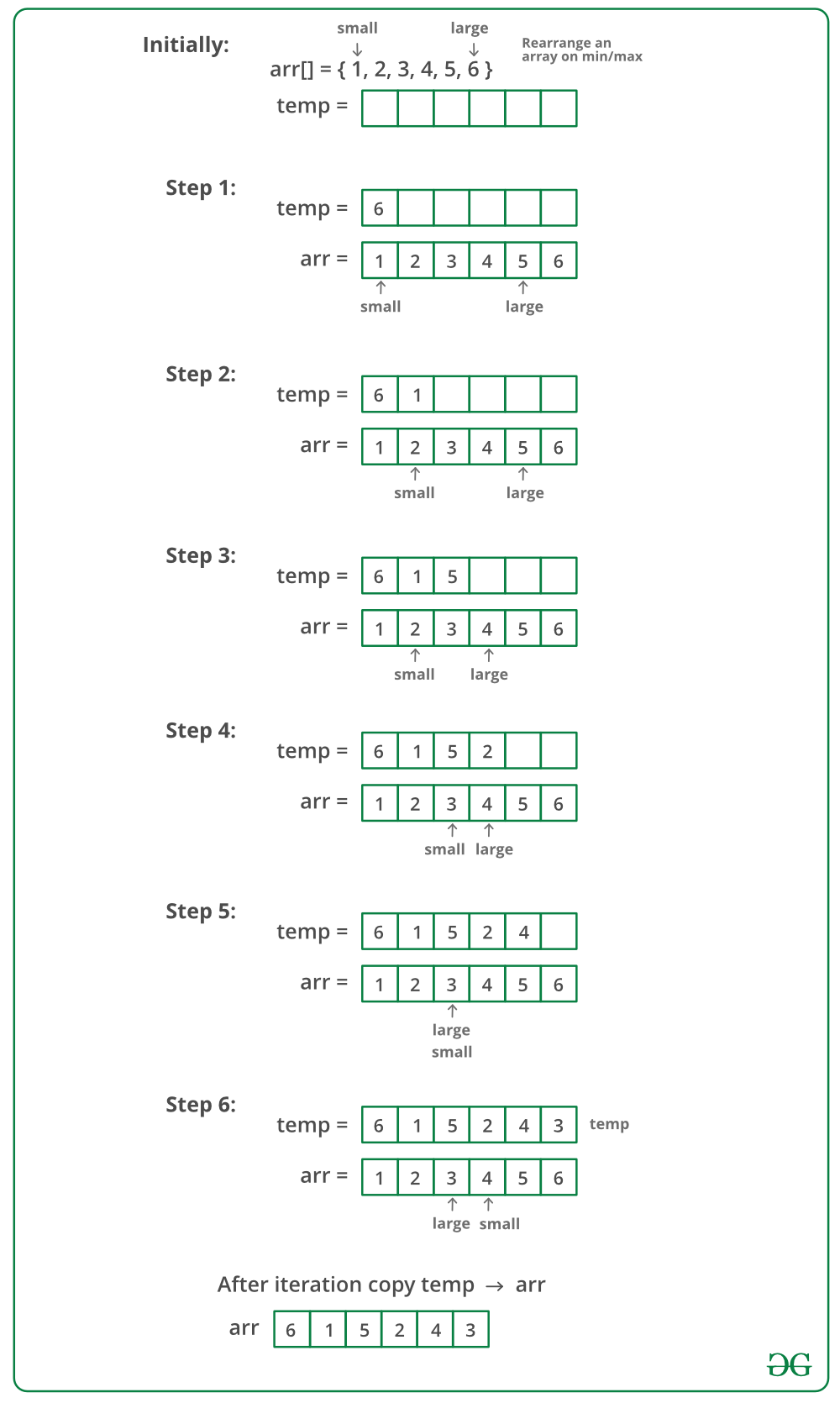# Rearrange an array in maximum minimum form | Set 1

• Difficulty Level : Medium
• Last Updated : 25 Aug, 2021

Given a sorted array of positive integers, rearrange the array alternately i.e first element should be maximum value, second minimum value, third second max, fourth second min and so on.

Examples:

Attention reader! Don’t stop learning now. Get hold of all the important DSA concepts with the DSA Self Paced Course at a student-friendly price and become industry ready.  To complete your preparation from learning a language to DS Algo and many more,  please refer Complete Interview Preparation Course.

In case you wish to attend live classes with experts, please refer DSA Live Classes for Working Professionals and Competitive Programming Live for Students.

Input: arr[] = {1, 2, 3, 4, 5, 6, 7}
Output: arr[] = {7, 1, 6, 2, 5, 3, 4}

Input: arr[] = {1, 2, 3, 4, 5, 6}
Output: arr[] = {6, 1, 5, 2, 4, 3}

Expected time complexity: O(n).

The idea is to use an auxiliary array. We maintain two pointers one to leftmost or smallest element and other to rightmost or largest element. We move both pointers toward each other and alternatively copy elements at these pointers to an auxiliary array. Finally, we copy the auxiliary array back to the original array.

Below image is a dry run of the above approach:Below is the implementation of the above approach:

## C++

 `// C++ program to rearrange an array in minimum``// maximum form``#include ``using` `namespace` `std;` `// Prints max at first position, min at second position``// second max at third position, second min at fourth``// position and so on.``void` `rearrange(``int` `arr[], ``int` `n)``{``    ``// Auxiliary array to hold modified array``    ``int` `temp[n];` `    ``// Indexes of smallest and largest elements``    ``// from remaining array.``    ``int` `small = 0, large = n - 1;` `    ``// To indicate whether we need to copy remaining``    ``// largest or remaining smallest at next position``    ``int` `flag = ``true``;` `    ``// Store result in temp[]``    ``for` `(``int` `i = 0; i < n; i++) {``        ``if` `(flag)``            ``temp[i] = arr[large--];``        ``else``            ``temp[i] = arr[small++];` `        ``flag = !flag;``    ``}` `    ``// Copy temp[] to arr[]``    ``for` `(``int` `i = 0; i < n; i++)``        ``arr[i] = temp[i];``}` `// Driver code``int` `main()``{``    ``int` `arr[] = { 1, 2, 3, 4, 5, 6 };``    ``int` `n = ``sizeof``(arr) / ``sizeof``(arr);` `    ``cout << ``"Original Array\n"``;``    ``for` `(``int` `i = 0; i < n; i++)``        ``cout << arr[i] << ``" "``;` `    ``rearrange(arr, n);` `    ``cout << ``"\nModified Array\n"``;``    ``for` `(``int` `i = 0; i < n; i++)``        ``cout << arr[i] << ``" "``;``    ``return` `0;``}`

## Java

 `// Java program to rearrange an array in minimum``// maximum form` `import` `java.util.Arrays;` `public` `class` `GFG``{``    ``// Prints max at first position, min at second position``    ``// second max at third position, second min at fourth``    ``// position and so on.``    ``static` `void` `rearrange(``int``[] arr, ``int` `n)``    ``{``        ``// Auxiliary array to hold modified array``        ``int` `temp[] = arr.clone();` `        ``// Indexes of smallest and largest elements``        ``// from remaining array.``        ``int` `small = ``0``, large = n - ``1``;` `        ``// To indicate whether we need to copy remaining``        ``// largest or remaining smallest at next position``        ``boolean` `flag = ``true``;` `        ``// Store result in temp[]``        ``for` `(``int` `i = ``0``; i < n; i++) {``            ``if` `(flag)``                ``arr[i] = temp[large--];``            ``else``                ``arr[i] = temp[small++];` `            ``flag = !flag;``        ``}``    ``}` `    ``// Driver code``    ``public` `static` `void` `main(String[] args)``    ``{``        ``int` `arr[] = ``new` `int``[] { ``1``, ``2``, ``3``, ``4``, ``5``, ``6` `};` `        ``System.out.println(``"Original Array "``);``        ``System.out.println(Arrays.toString(arr));` `        ``rearrange(arr, arr.length);` `        ``System.out.println(``"Modified Array "``);``        ``System.out.println(Arrays.toString(arr));``    ``}``}`

## Python

 `# Python program to rearrange an array in minimum``# maximum form` `# Prints max at first position, min at second position``# second max at third position, second min at fourth``# position and so on.`  `def` `rearrange(arr, n):``    ``# Auxiliary array to hold modified array``    ``temp ``=` `n``*``[``None``]` `    ``# Indexes of smallest and largest elements``    ``# from remaining array.``    ``small, large ``=` `0``, n``-``1` `    ``# To indicate whether we need to copy remaining``    ``# largest or remaining smallest at next position``    ``flag ``=` `True` `    ``# Store result in temp[]``    ``for` `i ``in` `range``(n):``        ``if` `flag ``is` `True``:``            ``temp[i] ``=` `arr[large]``            ``large ``-``=` `1``        ``else``:``            ``temp[i] ``=` `arr[small]``            ``small ``+``=` `1` `        ``flag ``=` `bool``(``1``-``flag)` `    ``# Copy temp[] to arr[]``    ``for` `i ``in` `range``(n):``        ``arr[i] ``=` `temp[i]``    ``return` `arr`  `# Driver code``arr ``=` `[``1``, ``2``, ``3``, ``4``, ``5``, ``6``]``n ``=` `len``(arr)``print``(``"Original Array"``)``print``(arr)``print``(``"Modified Array"``)``print``(rearrange(arr, n))` `# This code is contributed by Pratik Chhajer`

## C#

 `// C# program to rearrange``// an array in minimum``// maximum form``using` `System;` `class` `GFG {` `    ``// Prints max at first position,``    ``// min at second position second``    ``// max at third position, second``    ``// min at fourth position and so on.``    ``static` `void` `rearrange(``int``[] arr, ``int` `n)``    ``{``        ``// Auxiliary array to``        ``// hold modified array``        ``int``[] temp = ``new` `int``[n];` `        ``// Indexes of smallest``        ``// and largest elements``        ``// from remaining array.``        ``int` `small = 0, large = n - 1;` `        ``// To indicate whether we``        ``// need to copy remaining``        ``// largest or remaining``        ``// smallest at next position``        ``bool` `flag = ``true``;` `        ``// Store result in temp[]``        ``for` `(``int` `i = 0; i < n; i++) {``            ``if` `(flag)``                ``temp[i] = arr[large--];``            ``else``                ``temp[i] = arr[small++];` `            ``flag = !flag;``        ``}` `        ``// Copy temp[] to arr[]``        ``for` `(``int` `i = 0; i < n; i++)``            ``arr[i] = temp[i];``    ``}` `    ``// Driver Code``    ``static` `void` `Main()``    ``{``        ``int``[] arr = { 1, 2, 3, 4, 5, 6 };` `        ``Console.WriteLine(``"Original Array"``);``        ``for` `(``int` `i = 0; i < arr.Length; i++)``            ``Console.Write(arr[i] + ``" "``);` `        ``rearrange(arr, arr.Length);` `        ``Console.WriteLine(``"\nModified Array"``);``        ``for` `(``int` `i = 0; i < arr.Length; i++)``            ``Console.Write(arr[i] + ``" "``);``    ``}``}` `// This code is contributed``// by Sam007`

## PHP

 ``

## Javascript

 ``
Output
```Original Array
1 2 3 4 5 6
Modified Array
6 1 5 2 4 3 ```

Time Complexity: O(n)
Auxiliary Space: O(n)
https://youtu.be/pWASJvFqBW0?list=PLqM7alHXFySEQDk2MDfbwEdjd2svVJH9p
Exercise: How to solve this problem if extra space is not allowed?
Rearrange an array in maximum minimum form | Set 2 (O(1) extra space)